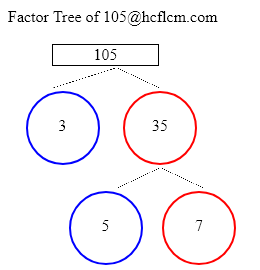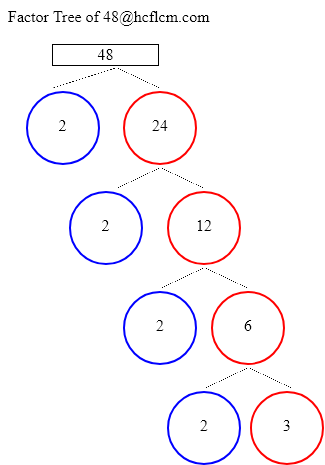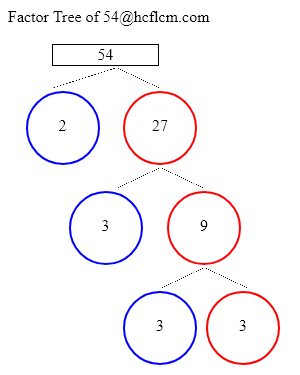Factor Tree Calculator

Factor Tree Calculator is an online tool that displays the factors of a given number. This online handy calculator makes the calculations easy and faster for you. Enter the number in the input field. Click on the 'calculate' button and you will get the factors. It Displays the factors in a fraction of seconds.

Factor Tree of:

Factor Tree Examples:

Related Calculators:

What is Factor Tree?

Usually, Factors of a number are defined as the numbers which are multiplied together to find the original number. In general Factor Tree is a tool that breaks down numbers into prime numbers. Usually, numbers expressed at the end in a Factor Tree are the prime factors of a given number. For instance, factors of a number 56 are 2 x 2 x 2 x 7.

How to find Factor Tree of a number?

Follow the simple steps prevailing over here to get the Factor Tree and it applies to any nonprime number.

• Firstly, find 2 factors of a number.
• Just have a look at the two factors and determine if at least one of them is not prime.
• If it is not a prime number continue the process further.
• Make sure you do the process until all the numbers are prime.

Once you get the number multiples of prime numbers that is called the Factor Tree.

How to use Factor Tree Calculator?

Step 1: Enter the number in the input field.

Step 2: Click on the button and you will get the factors.

Step 3: Factors will be displayed in the output field.hcflcm.com online factor tree calculator performs the complex calculation faster, and it showcases the factors in a fraction of seconds.

Factor Tree Method Examples

Example 1: Find the Factor Tree of 105?

Solution:105 = 3 x 35

35 = 5 x 7

If we write into multiples it would be 105 x 3

On splitting 35 further and writing it as multiples of numbers it would be 7 x 5.

Altogether expressing the number in terms of prime factors would be 3 x 5 x 7.

Example 2: Find the Factor Tree of 48?

Solution:48 = 2 x 24

24 = 2 x 12

12 = 2 x 6

6 = 2 x 3

If we write into multiples it would be 48 x 2

On splitting 24 further and writing it as multiples of numbers it would be 12 x 2.

On splitting 12 further and writing it as multiples of numbers it would be 6 x 2.

On splitting 6 further and writing it as multiples of numbers it would be 3 x 2.

Altogether expressing the number in terms of prime factors would be 2 x 2 x 2 x 2 x 3.

Example 3: Find the Factor Tree of 54?

Solution:54 = 2 x 27

27 = 3 x 9

9 = 3 x 3

If we write into multiples it would be 54 x 2

On splitting 27 further and writing it as multiples of numbers it would be 9 x 3.

On splitting 9 further and writing it as multiples of numbers it would be 3 x 3.

Altogether expressing the number in terms of prime factors would be 2 x 3 x 3 x 3.

FAQs on Factor Tree Calculator?

1. How to find a Factor Tree of numbers?

You can find the Factor Tree of a number easily by simply giving the inputs on our calculator and you will get the output at one go.

2. Where can I get the Factor Tree Concept along with Examples?

You can get all the concepts related to Factor Tree with clear explanation using examples on our page. Get to know the concepts in a much better way using the info on our page.

3. What is meant by Factor Tree of number?

Numbers expressed as the multiplies of prime numbers is called Factor Tree.